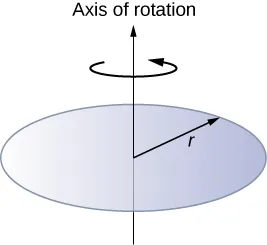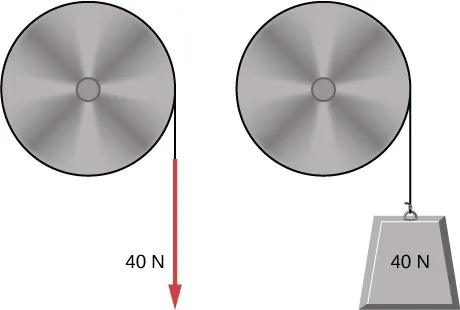University Physics Volume 1

# Challenge Problems

University Physics Volume 1Challenge Problems

### Challenge Problems

121.

The angular acceleration of a rotating rigid body is given by $α=(2.0−3.0t)rad/s2α=(2.0−3.0t)rad/s2$. If the body starts rotating from rest at $t=0t=0$, (a) what is the angular velocity? (b) Angular position? (c) What angle does it rotate through in 10 s? (d) Where does the vector perpendicular to the axis of rotation indicating $0°0°$ at $t=0t=0$ lie at $t=10st=10s$?

122.

Earth’s day has increased by 0.002 s in the last century. If this increase in Earth’s period is constant, how long will it take for Earth to come to rest?

123.

A disk of mass m, radius R, and area A has a surface mass density $σ=mrARσ=mrAR$ (see the following figure). What is the moment of inertia of the disk about an axis through the center?124.

Zorch, an archenemy of Rotation Man, decides to slow Earth’s rotation to once per 28.0 h by exerting an opposing force at and parallel to the equator. Rotation Man is not immediately concerned, because he knows Zorch can only exert a force of $4.00×107N4.00×107N$ (a little greater than a Saturn V rocket’s thrust). How long must Zorch push with this force to accomplish his goal? (This period gives Rotation Man time to devote to other villains.)

125.

A cord is wrapped around the rim of a solid cylinder of radius 0.25 m, and a constant force of 40 N is exerted on the cord shown, as shown in the following figure. The cylinder is mounted on frictionless bearings, and its moment of inertia is $6.0kg·m26.0kg·m2$. (a) Use the work energy theorem to calculate the angular velocity of the cylinder after 5.0 m of cord have been removed. (b) If the 40-N force is replaced by a 40-N weight, what is the angular velocity of the cylinder after 5.0 m of cord have unwound?Order a print copy

As an Amazon Associate we earn from qualifying purchases.